Download Presentation# Business Finance - PowerPoint PPT Presentation

Business Finance. BA303 Michael Dimond. Module G: Financial Statement Analysis. Understanding financial statements. Balance Sheet Income Statement Statement of Cash Flows Statement of Shareholders’ Equity. Meaningful Ratio Analysis.I am the owner, or an agent authorized to act on behalf of the owner, of the copyrighted work described.
Download PresentationAn Image/Link below is provided (as is) to download presentation

Download Policy: Content on the Website is provided to you AS IS for your information and personal use and may not be sold / licensed / shared on other websites without getting consent from its author.While downloading, if for some reason you are not able to download a presentation, the publisher may have deleted the file from their server.

- - - - - - - - - - - - - - - - - - - - - - - - - - E N D - - - - - - - - - - - - - - - - - - - - - - - - - -
Presentation TranscriptBA303

Michael Dimond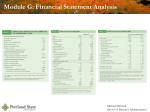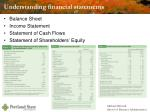Understanding financial statements
• Balance Sheet
• Income Statement
• Statement of Cash Flows
• Statement of Shareholders’ Equity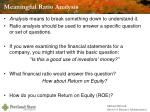Meaningful Ratio Analysis
• Analysis means to break something down to understand it.
• Ratio analysis should be used to answer a specific question or set of questions.
• If you were examining the financial statements for a company, you might start with this basic question:

“Is this a good use of investors’ money?”

• What financial ratio would answer this question?

How about Return on Equity?

• How do you compute Return on Equity (ROE)?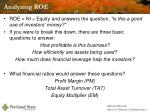Analyzing ROE
• ROE = NI ÷ Equity and answers the question, “is this a good use of investors’ money?”
• If you were to break this down, there are three basic questions to answer:

How profitable is this business?

How efficiently are assets being used?

How much does financial leverage help the investors?

• What financial ratios would answer these questions?

Profit Margin (PM)

Total Asset Turnover (TAT)

Equity Multiplier (EM)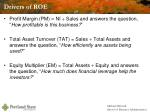Drivers of ROE
• Profit Margin (PM) = NI ÷ Sales and answers the question, “How profitable is this business?”
• Total Asset Turnover (TAT) = Sales ÷ Total Assets and answers the question, “How efficiently are assets being used?”
• Equity Multiplier (EM) = Total Assets ÷ Equity and answers the question, “How much does financial leverage help the investors?”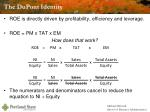The DuPont Identity
• ROE is directly driven by profitability, efficiency and leverage.
• ROE = PM x TAT x EM

How does that work?

• The numerators and denominators cancel to reduce the equation to NI ÷ EquityA note about the text’s version of ROE & DuPont
• The author uses Earnings Available to Common Shareholders for ROE computations. While this is not terribly incorrect, it isn’t really correct either.
• Net Income ÷ Equity = ROE
• Earnings Available to Common Shareholders ÷ (Equity – Preferred Equity) = Return on Common Equity (ROCE, not ROE)
• The purpose of analysis is to answer important questions.
• If the question is how hard the investors’ money is working, compute ROE
• To find how hard common shareholders’ money is working, compute ROCE
• Never mix & match. ROCE uses Earnings Available to Common Shareholders and Common Equity. ROE uses Net Income and Total Equity.
• Notice the author computes ROA as Earnings Available to Common Shareholders ÷ Total Assets. What is wrong with this?
• ROE & ROA are sometimes very manipulated figures, used by managers to prove a point. Always compute your own figures for analysis.A word about ROA
• ROA = Return on Assets
• What’s the difference between Equity & Assets?
• Leverage
• What’s the difference between ROE & ROA?
• Leverage
• ROE = PM x TAT x EM
• EM represents leverage
• ROA = PM x TAT
• No leverageDigging Deeper with Financial Ratios
• How would you analyze profitability, efficiency and leverage?
• How do profitability, efficiency and leverage relate?
• What affects profitability?
• What drives sales?
• What is the composition of assets?
• How were assets paid for?
• How are liabilities managed?
• Where shall we begin?Common-Size Financial Statements
• Shows each line item as a percent of an appropriate total.
• Common-size balance sheet
• % of Total Assets
• Shows the composition of assets
• Liabilities & equity items are also shown as % of total assets
• Debt Ratio = Total Liabilities ÷ Total Assets
• Common-size income statement
• % of Sales
• PM = Net Income as % of SalesWe don’t make a common-size CF Statement

There are other ways to examine relevant information which would be more helpfulVertical & Horizontal Analysis
• Vertical Analysis compares figures as a percent of a relevant total (“common size” financial statements)
• Horizontal Analysis compares the same figure over a series of periods (showing % change or % growth)Measuring growth
• Financial figures change from year to year
• To find the % change (“% growth”) over a 1-year period, divide the difference of the two figures by the first year’s value:
• [ending – beginning] / [beginning]

OR

• [ending] / [beginning] - 1
• Measuring growth over more than one period means we need to find the average growth during that time.CAGR: Compound Annual Growth Rate
• The CAGR is the result of compounded increase over time at a specific average rate
• (133.1/100)^(1/3)-1=0.10
• It can be tested by plugging the result into a compounding formula using the same figures
• 100*(1+0.10)^3=133.10
• It can be figured using the TVM functions on your calculator
• PV = -100 FV = 133.10 n = 3 PMT = 0 solve for I = 10%
• What if you were given a series of % changes instead of dollar figures?
• Year 1: 10% increase, Year 2: 12% increase, Year 3: 8% increase
• You need to find the Geometric Average Growth over the three year periodGeometric Average vs Arithmetic Average
• Arithmetic Average only shows the “typical” result
• Geo Avg = [(1+20%)*(1+-16.67%)* (1+20%)*(1+16.67%)]^(1/4) -1 = 8.78%
• CAGR also shows the result of compounding
• (14/10)^(1/4) – 1 = 0.878 = 8.78%
• The price didn’t increase 8.78% each year, but we end up with the same final value if we compound it by 8.78% every year.
• 5 years means 4 periods of compounding, so we find the 4th root ( ^1/4 power)Categories of Financial Ratios
• Most finance texts group ratios into categories like these:
• Profitability ratios
• Efficiency (or Activity) ratios
• Liquidity ratios
• Debt ratios
• Market ratios
• It is usually more helpful to think of the questions to be answered rather than just crunching a bunch of numbers.
• Uses critical thinking
• Easier to read
• Less time consuming
• Uses fewer resourcesProfitability Ratios
• PM = Net Income ÷ Sales (Sometimes called “Net Profit Margin”).
• This also is the bottom line on a common-size income statement
• The author makes a distinction for Earnings Available to Common Shareholders.
• Gross Margin = Gross Profit ÷ Sales
• Gross Profit = Sales – COGS
• Also called the “Gross Profit Margin”
• Operating Margin = Operating Profit ÷ Sales
• Also called the “Operating Profit Margin”Efficiency Ratios
• TAT = Sales ÷ Total Assets
• How hard do specific assets work?
• Inventory Turnover
• Inventory Turnover = Sales ÷ Inventory
• The label “Inventory Turnover” is also used for COGS ÷ Avg. Inventory
• These two ratios answer different questions:
• How hard is inventory working? (Sales/Inventory)
• How many times/year is inventory replaced? (COGS/Average Inventory)
• How would you convert this into “Days in Inventory?”
• Average Collection Period or AR Conversion Period
• Days to Collect AR = Avg. Accts Receivable ÷ Avg. Daily Sales
• Average Daily Sales = Sales ÷ 365
• The sales figure should exclude sales paid for in cash, use only sales creating AREfficiency Ratios
• Average Payment Period
• Days to Pay AP = Avg. AP ÷ Avg Daily Purchases
• Avg Daily Purchases = Purchases ÷ 365
• Purchases = COGS + Ending Inventory – Beginning Inventory
• If you know how long it takes a company to sell inventory, how long it takes to collect accounts receivable and how long to pay its bills, you can compute how long their business takes to function
• Operating Cycle: Days in Inventory + Days in Receivables
• Cash Cycle: Days in Inventory + Days in Receivables – Days in PayablesEfficiency Ratios
• There is an easy and consistent way to compute and understand the components of the cash cycle.
• Each of the “Days in…” figures represents a year divided by the appropriate turnover rate:
• Days in Inventory = 365 ÷ Inventory Turnover Rate
• Days in Receivables = 365 ÷ Receivables Turnover Rate
• Days in Payables = 365 ÷ Payables Turnover Rate
• This means the turnover rates can be simplified to these:
• Inventory Turnover Rate = COGS ÷ Avg. Inventory
• Receivables Turnover Rate = Sales ÷ Avg. Receivables
• Payables Turnover Rate = Purchases ÷ Avg. Payables
• …and the days in each can be computed as:
• Days in Inventory = 365 ÷ (COGS ÷ Avg. Inventory)
• Days in Receivables = 365 ÷ (Sales ÷ Avg. Receivables)
• Days in Payables = 365 ÷ (Purchases ÷ Avg. Payables)Liquidity Ratios
• The Current Ratio
• Pretty much useless in my opinion, but memorize it anyway.
• Current Assets ÷ Current Liabilities
• Liquidity means something can be converted into cash immediately without significant loss of value. Current Assets includes inventory. Is inventory really liquid?
• Quick Ratio (also called the “Acid Test”)
• Answers the question, “how well can this firm meet its short-term obligations?”
• [Current Assets – Inventory] ÷ Current LiabilitiesDebt Management Ratios
• Debt ratio = Total Liabilities ÷ Total Assets
• Also called “Debt to Total Capital” ratio
• Debt-to-Equity ratio = Total Liabilities ÷ Total Equity
• EM (from DuPont) = 1 + D/E
• Times Interest Earned ratio = EBIT ÷ Interest
• NOTE: The book has a typo on page 78. It claims TIE = EBIT ÷ Tax, which is not correct.
• TIE can be altered to cover any financial obligations.
• TIE = EBIT ÷ Interest
• :. TIE = (EBT + Interest) ÷ Interest
• (EBT + Interest + Lease Pmts) ÷ (Interest + Lease Pmts) = Fixed Payment Coverage
• (EBT + Interest + Lease Pmts) ÷ (Interest + Lease Pmts + Principal Repayments/(1-t)) = Fixed Payment CoverageMarket Value Ratios
• Price-to-Earnings ratio = Share Price ÷ Earnings per Share
• Earnings per Share (EPS) = Earnings Available to Common Shareholders ÷ Number of Shares of Common Stock
• If there is no preferred equity (or an insignificant amount), EPS can be NI ÷ Number of Shares
• Because the Numerator and Denominator are both “per share,” the PE ratio can be computed as Market Capitalization ÷ Total Earnings Available
• Market-to-Book ratio = Price per Share ÷ Book Value per Share
• Book Value per Share = Common Equity on Balance Sheet ÷ Number of Shares
• Common Equity = All equity except preferred equity
• Again, because the Numerator and Denominator are both “per share,” the MB ratio can be computed as Market Capitalization ÷ Total Common EquityOther useful analysis
• Dividends & Retained Earnings
• d: Dividend Payout Ratio = Dividends ÷ Net Income
• b: Retention Ratio = 1 – d

Also called the “plowback ratio.” Why do you think that name is used?

• Growth Limitations
• SGR: Sustainable Growth Rate = b x ROE = b x PM x TAT x EM
• IGR: Internal Growth Rate = b x ROA = b x PM x TAT
• Breakeven
• BE = Total Fixed Costs ÷ Contribution Margin
• Contribution Margin = Price per unit – Variable Costs per unit
• Operating, Accounting, and Financial breakevens all exist. The definition of “Fixed Costs” changes.
• Degree of Operating Leverage
• Looks a lot like an elasticity formula: %Δ Op. Income ÷ %ΔSales
• As firm approaches breakeven, DOL gets larger
• A point estimate of DOL can be computed as Gross Profit ÷ Operating Income